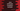# Java ArithmeticException explanation with examples## Java ArithmeticException explanation with examples:

The ArithmeticException is an exception that is thrown by an arithmetic condition. For example, if we are trying to divide a number by zero, it will throw an ArithmeticException.

In this post, I will show you how ArithmeticException works with examples.

### ArithmeticException class:

The full package of ArithmeticException is java.lang.ArithmeticException. Its parent class is java.lang.RuntimeException and the parent class of RuntimeException is java.lang.Exception.

As you can see that it is a RuntimeException. So, the compiler will not show any error.

### Constructors of ArithmeticException:

Following are the constructors of ArithmeticException:

``````ArithmeticException()

ArithmeticException(String s)``````

The first one will create one object of ArithmeticException without any detailed message and the second one will create one ArithmeticException object with a detailed message.

### Example: Dividing by zero:

This is a common cause of ArithmeticException. If you try to divide a number by zero, it will throw an ArithmeticException.

For example:

``````public class Main {
public static void main(String[] args) {
System.out.println(10/0);
}
}``````

As this is a RuntimeException, the compiler will not show any error and we can run the program. It will throw the following error:

``````Exception in thread "main" java.lang.ArithmeticException: / by zero
at com.company.Main.main(Main.java:5)``````

### How to handle ArithmeticException:

We can use a try-catch block to handle ArithmeticException:

``````public class Main {
public static void main(String[] args) {
try {
System.out.println(10 / 0);
} catch (ArithmeticException e) {
System.out.println("ArithmeticException occurred: " + e.getMessage());
}
}
}``````

In the catch block, we can log this exception or we can add a meaningful message. In this example, I have added a message with the return value of getMessage(). The getMessage() method returns the detailed message string of the exception.